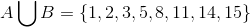## Example Questions

2 Next →

### Example Question #1 : How To Find The Union Of A Venn Diagram

Forty students play soccer and/or basketball after school. Twenty-four students play soccer and twenty-nine play basketball. How many students play both soccer and basketball?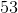Explanation:

We can draw a Venn diagram of these students.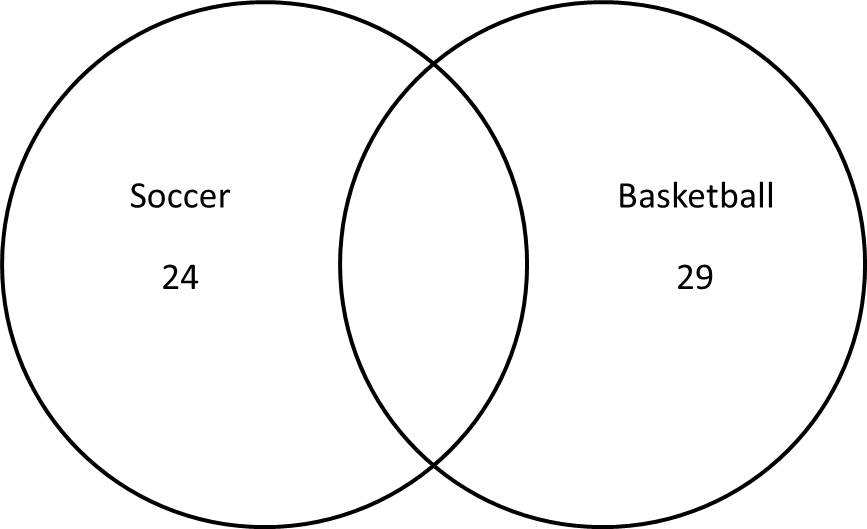Drawn this way, there are more students on the Venn diagram than we have.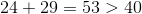This is because some of the students play both sports and should be in the overlap on the Venn diagram. To find the number of students in the overlap, subtract the total number of students given from the number on the diagram.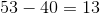This represents the number of students who were counted twice, or the number in the overlap.

We can redraw the correct Venn diagram with this number.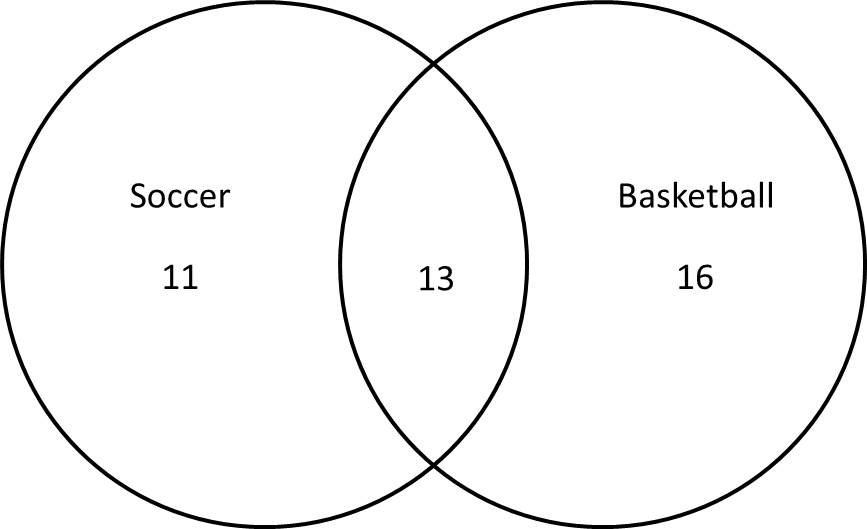### Example Question #11 : Venn Diagrams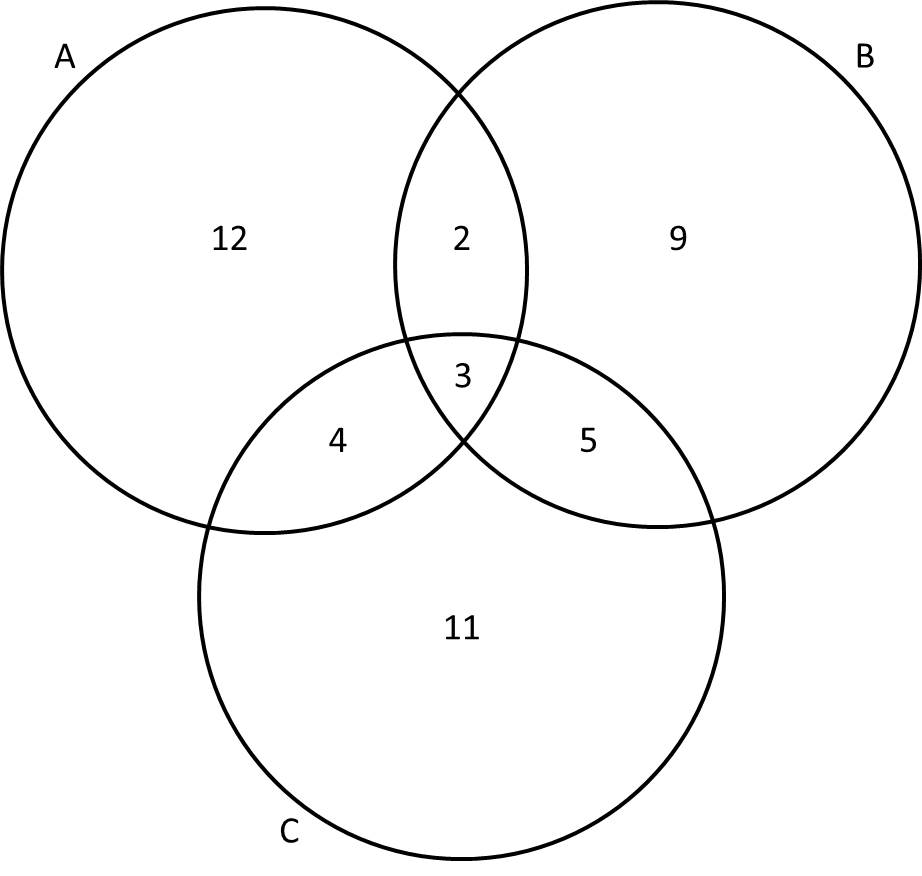Giving the Venn diagram above, what is the sum of the numbers in the set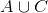?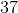Explanation:

The notationstands for "A union C," which refers to everything that is in either setor set.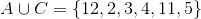When we add the numbers together, we get: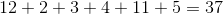### Example Question #11 : Venn Diagrams

In a class of senior high-school students,have pet cats,have pet dogs,have both cats and dogs, andhave neither cats nor dogs. How many total students are in the class?Explanation:

A Venn diagram can help us determine the total number of students in the class.

First, we must calculate the number of students who have ONLY cats or ONLY dogs. First, for cats, 15 students have cats, and 5 students have both cats and dogs.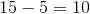Ten students have only cats.

For dogs, 12 students have dogs, and 5 students have both cats and dogs.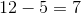Seven students have only dogs.

Using this information, we can fill in the Venn diagram.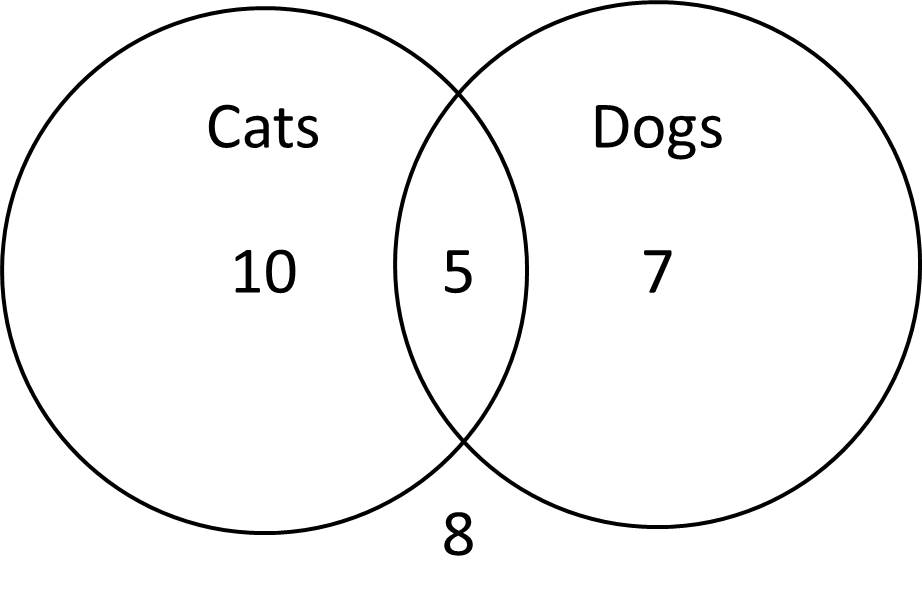This diagram shows the 10 students with only cats, the 7 students with only dogs, the 5 students with both, and the 8 students with neither.  Adding up the numbers will give us the total number of students.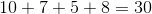### Example Question #11 : Venn Diagrams

Let Set A =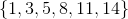and Set B =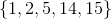.

What is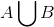?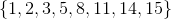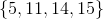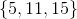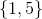represents the union of the two sets. The union of Sets A and B is the set of elements which appear in A, in B, or in both A and B.
Therefore,